#### You may also like### Integral Equation

Solve this integral equation.### Integral Sandwich

Generalise this inequality involving integrals.### Integral Inequality

An inequality involving integrals of squares of functions.

# Area L

##### Age 16 to 18 Challenge Level:
We received solutions from Amrit, Adithya, Guruvignesh and Agathiyan, all from Hymers College, and Pablo from Kings College Alicante. Here is Pablo's solution: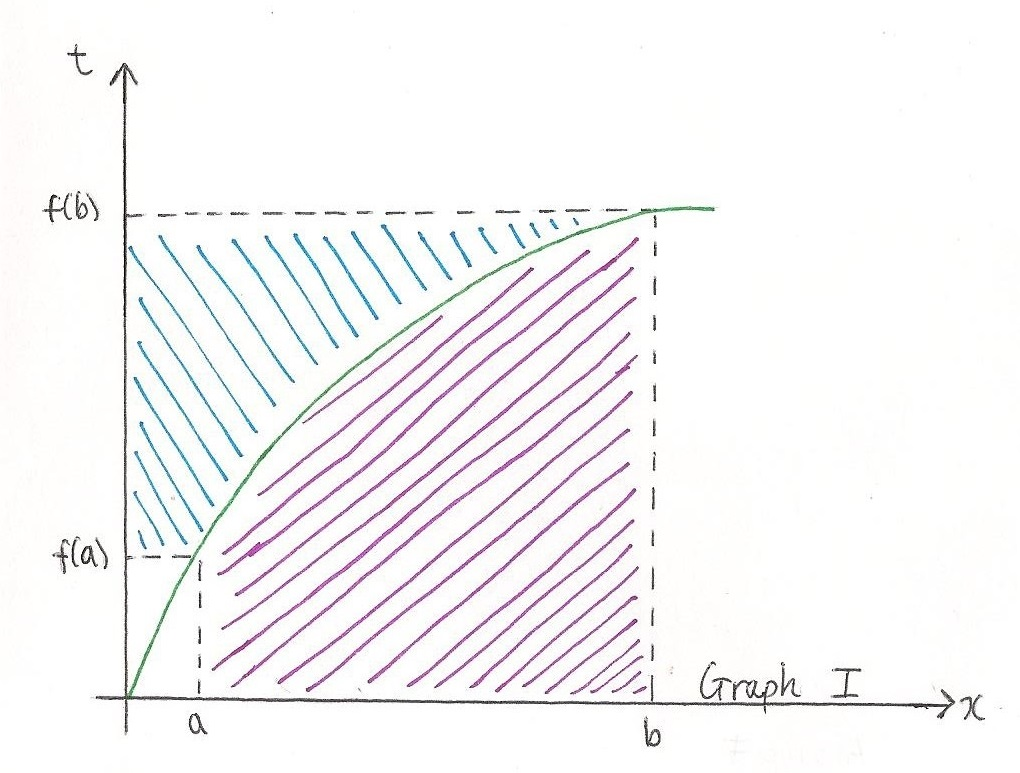As you can see in Graph I, the areas in purple and blue add up to the big rectangle, $bf(b)$, minus $af(a)$, the small rectangle.

There is a change of variable in $f^{-1}(t)$ because the area isn't taken
from the x-axis, but from the t-axis. $f^{-1}(x)$ is the inverse of $f(x)$. It is obtained either by switching variables and rearranging the equation again or by reflecting it in the line y=x.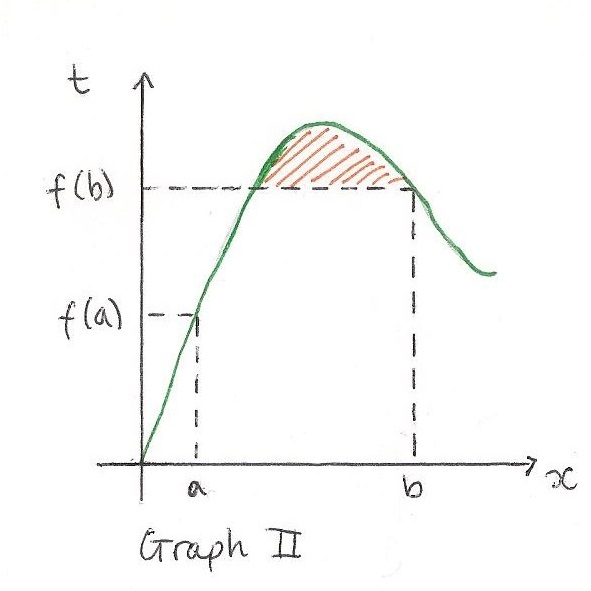The reason why $f(x)$ must be increasing in the interval  $a \leq x \leq b$ is shown in Graph II. If it weren't, if at some point it reached a turning point and began to decrease, the area (orange) at the top would not be accounted for.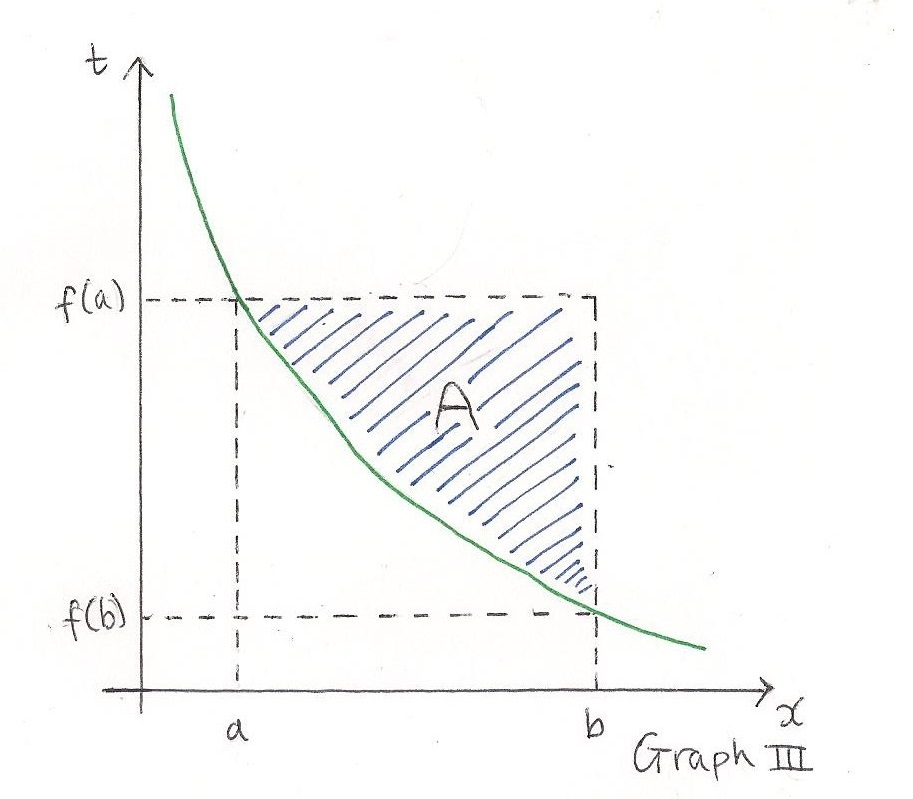If $f(x)$ is always decreasing in the interval  $a \leq x \leq b$, a similar formula comes out, but through more algebraic manipulation. The area called A in Graph III is:

$A = bf(a) - bf(b) - \int_{f(b)}^{f(a)} f^{-1}(t) d t$

but also, $A = bf(a) - af(a) \int_a^b f(x) d x$

so $$bf(a) - bf(b) - \int_{f(b)}^{f(a)} f^{-1}(t) d = bf(a) - af(a) \int_a^b f(x) d x$$ $$\int_a^b f(x) d x = bf(a) - af(a) - \left[bf(a) - bf(b) - \int_{f(b)}^{f(a)} f^{-1}(t) d t\right]$$ $$\int_a^b f(x) d x = bf(b) - af(a) + \int_{f(b)}^{f(a)} f^{-1}(t) d t$$

The difference with the first formula is that $\int_{f(b)}^{f(a)} f^{-1}(t) d t$ is added, not subtracted. Additionally, the boundaries are swapped, as $f(b)$ is less than $f(a)$.

First, we can find the value of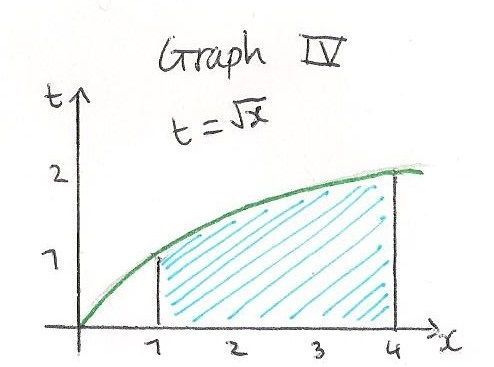$\int_1^4\sqrt t dt$ the conventional
way:
\eqalign{\int_1^4 \sqrt x dx &= \left[\frac23 x^\frac32\right]_1^4 \\ &= \frac23 ( 4^\frac32 - 1^\frac32) \\ &= \frac{14}3}

Now using our formula:

\eqalign{\int_1^4 \sqrt x dx &= bf(b) - af(a) - \int_{f(a)}^{f(b)}f^{-1}(t)dt \\ &= 4\sqrt4 - 1\sqrt1 - \int_{\sqrt(1)}^{\sqrt(4)} t^2 dt \\ &= 8 - 1 - \left[\frac13 t^3 \right]_1^2 \\ &= 7 - \frac83 + \frac13 \\ &= \frac{14}3}

For $\sin^{-1}(x)$, using Graph V: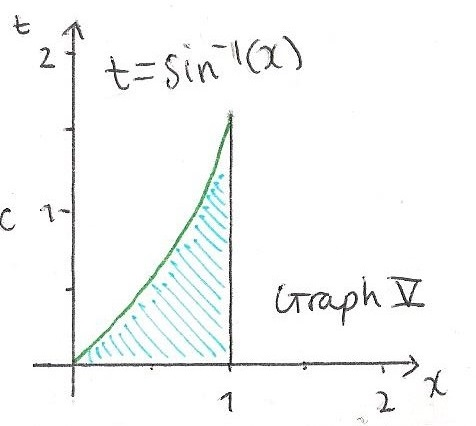\eqalign{ \int_0^1 \sin^{-1}(x) dx &= bf(b) - af(a) - \int_{f(a)}^{f(b)}f^{-1}(t)dt \\ &= 1\times \sin^{-1}(1) - 0\times \sin^{-1}(0) - \int_{f(a)}^{f(b)} \sin(t) dt \\ &= \frac{\pi}2 - 0 - \int_0^{\frac{\pi}2} \sin(t) dt \\ &=\frac{\pi}2 - \left[-\cos(t)\right]_0^{\frac{\pi}2} \\ &= \frac{\pi}2 - \left[(-cos(\frac{\pi}2)-(-cos(0))\right]\\ &= \frac{\pi}2 - 1}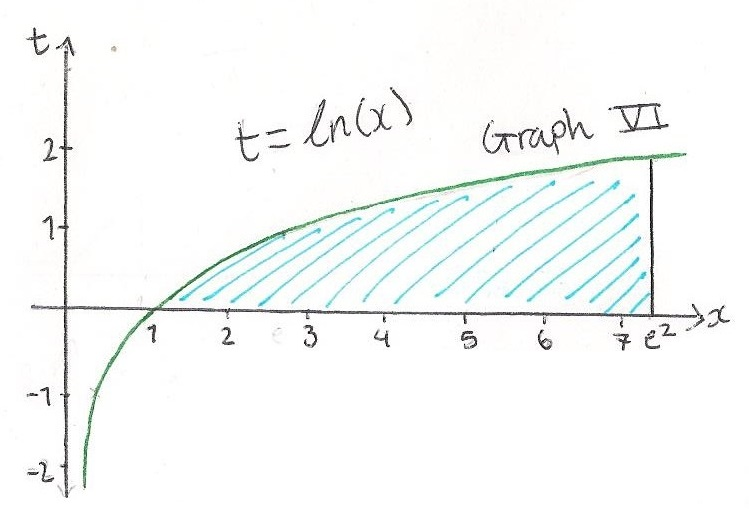Another function that can be integrated using this method is $ln(x)$:
\eqalign{\int_1^{e^2} ln(x) dx &= e^2ln(e^2) - 1ln(1) - \int_0^2 e^t dt \\ &= 2e^2 - 0 - \left[e^t\right]_0^2 \\ &= 2e^2 - (e^2 - e^0) \\ &= 2e^2 - e^2 + 1 \\ &= e^2 + 1}